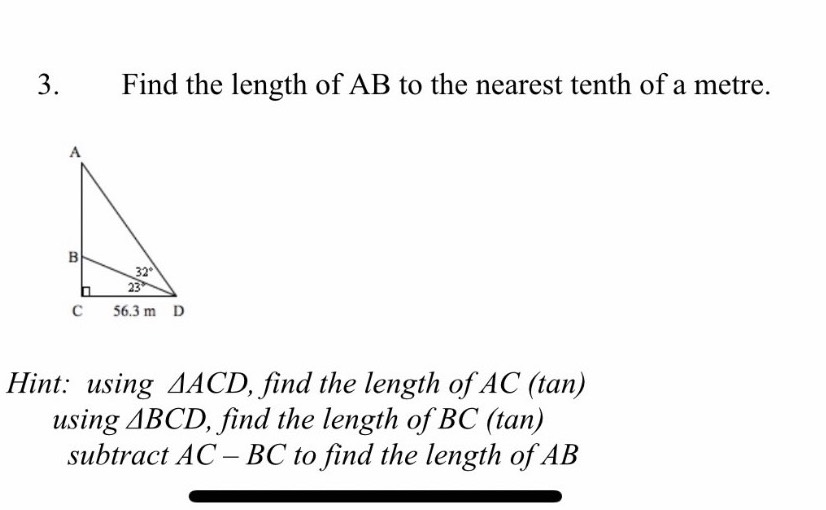### Still have math questions?Find the length of AB to the nearest tenth of a metre. Hint: using $$\triangle A C D$$ , find the length of $$A C$$ (tan) using $$\triangle B C D$$ , find the length of $$B C$$ (tan) subtract $$A C - B C$$ to find the length of $$A B$$